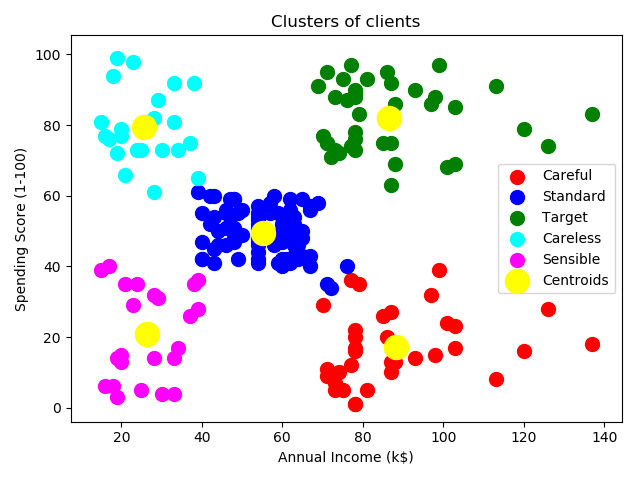# 机器学习A-Z～K平均聚类算法

## 原理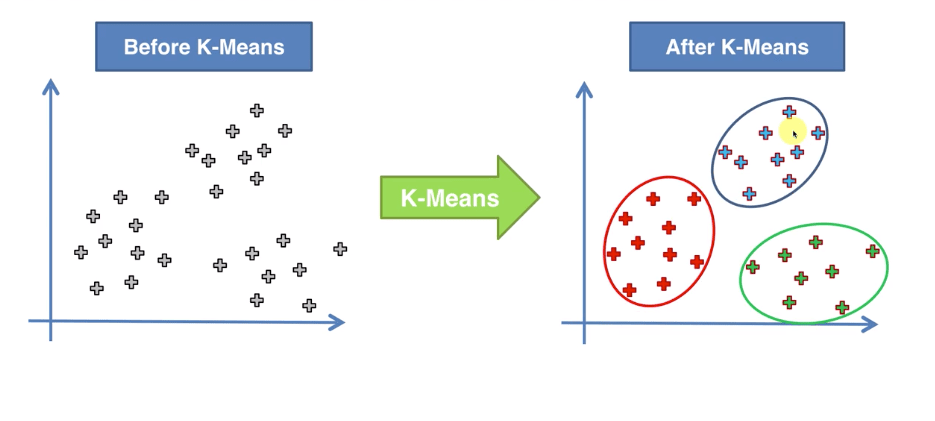1. 选择我们想要的类的个数K；
2. 在平面上随机选择K个点，作为初始化类的中心点，不一定在原先数据当中；
3. 对于数据集中的每个点，要判断它属于我们之前K个中心点的哪一类。依据数据中的每个点对这K个点的距离的大小，找到最短的距离，那么就是每个数据点对应的类别，这一步可以称作是分配；
4. 重新计算一些新的中心点，就是应用之前分配的结果重新计算分配好的每个类当中的中心点；
5. 重新分配，如果重新分配的结果和之前分配的结果相同，则说明找到最佳的K-Means算法的结果，如果不同，那继续去第四步进行分配计算，直到找到最佳算法结果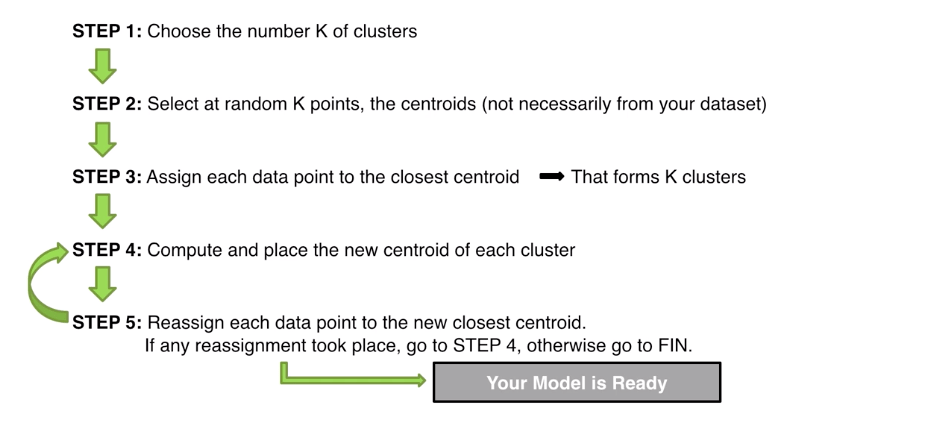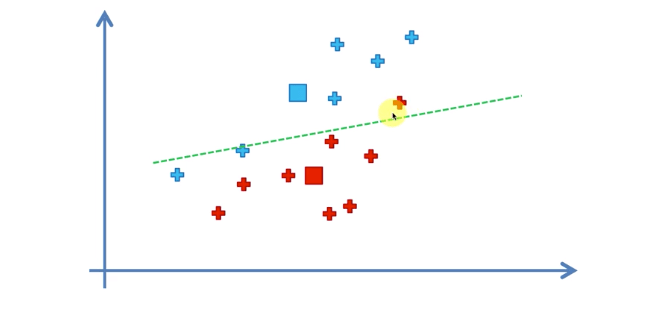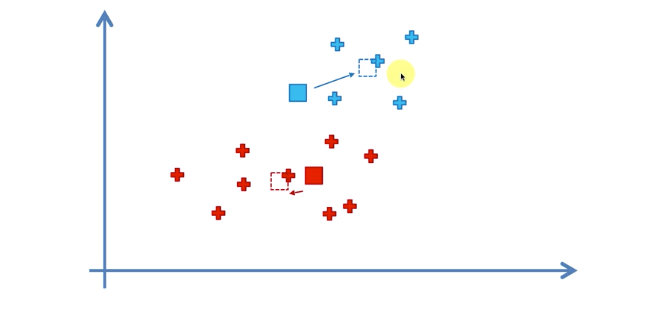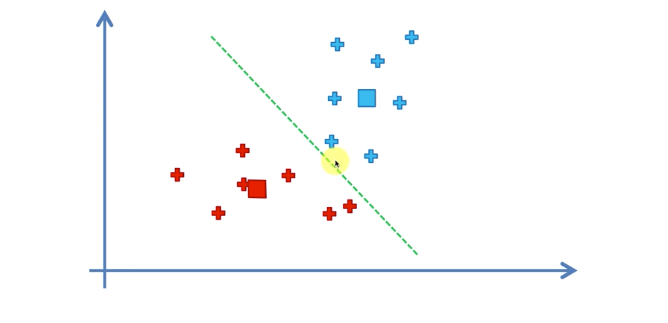K-Means算法可以以一种算法的方式告诉我们最佳的聚类的方式，这里就得到了左下方红组，右上方蓝组的这样一个结论。

## 随机初始化陷阱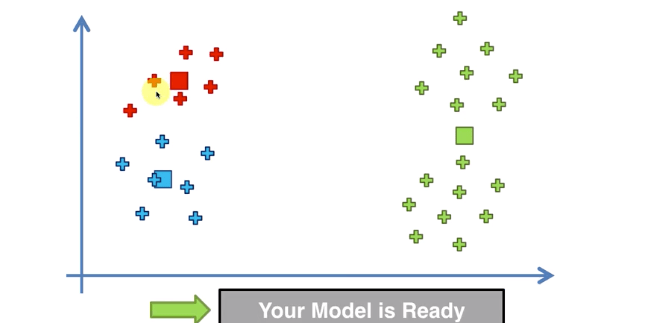## 选择类的个数### 代码实现

CustomerID Genre Age Annual Income (k$) Spending Score (1-100) 0001 Male 19 15 39 0002 Male 21 15 81 0003 Female 20 16 6 0004 Female 23 16 77 那么这个问题的自变量就是第三四列，年收入和购物指数。但它是个无监督学习，因此没有因变量。这里我们要用到的工具是sklearn.cluster中的KMeans类。 首先要计算各个分组的WCSS。这里我们计算组数从1到10的情况。 wcss = [] for i in range(1, 11): kmeans = KMeans(n_clusters=i, max_iter=300, n_init=10, init='k-means++', random_state=0) kmeans.fit(X) wcss.append(kmeans.inertia_) plt.plot(range(1, 11), wcss) plt.title('The Elbow Method') plt.xlabel('Number of Clusters') plt.ylabel('WCSS') plt.show()  这里的KMeans中的参数也解释下，n_clusters指的是分组数，max_iter指的是每一次计算时最大的循环个数，这里使用默认值300，n_init代表每一个做K平均算法时，会对多少组不同的中心值进行计算。init这个参数非常重要，指的是我们如何选择初始值，最简单的是random，即随机，但为了避免掉入随机初始值陷阱，这里使用k-means++。 拟合好后得到组间距离就是kmeans.inertia_。这样我们就可以画出对于不同的分组数，wcss的图像。那么通过手肘法则，可以得到最佳的分组个数是5组，则可以开始拟合数据。 # Applying the k-means to the mall dataset kmeans = KMeans(n_clusters=5, max_iter=300, n_init=10, init='k-means++', random_state=0) y_kmeans = kmeans.fit_predict(X)  拟合好数据后，得到的y_means实际上就是0-4五个分组。我们来将分组后的图像画出来看看。 # Visualizing the clusters plt.scatter(X[y_kmeans == 0, 0], X[y_kmeans == 0, 1], s=100, c='red', label='Careful') plt.scatter(X[y_kmeans == 1, 0], X[y_kmeans == 1, 1], s=100, c='blue', label='Standard') plt.scatter(X[y_kmeans == 2, 0], X[y_kmeans == 2, 1], s=100, c='green', label='Target') plt.scatter(X[y_kmeans == 3, 0], X[y_kmeans == 3, 1], s=100, c='cyan', label='Careless') plt.scatter(X[y_kmeans == 4, 0], X[y_kmeans == 4, 1], s=100, c='magenta', label='Sensible') plt.scatter(kmeans.cluster_centers_[:, 0], kmeans.cluster_centers_[:, 1], s=300, c='yellow', label='Centroids') plt.title('Clusters of clients') plt.xlabel('Annual Income (k$)')
plt.ylabel('Spending Score (1-100)')
plt.legend()
plt.show()